Next: Edit Scripts Up: Overview and Preliminaries Previous: Overview and Preliminaries

## Edit Operations

In an ordered tree, if nodes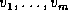are the children of node u, then we call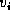the ith child of u. For a node x, we let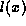denote the label of x,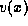denote the value of x, and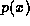denote the parent of x if x is not the root. We assume that labels are chosen from a fixed but arbitrary set. In the definitions of the edit operations,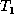refers to the tree on which the operation is applied, while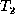refers to the resulting tree. The four edit operations on trees are the following:

Insert:
The insertion of a new leaf node x into, denoted by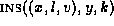. A node x with label l and value v is inserted as the kth child of node y of. More precisely, if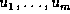are the children of y in, then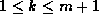and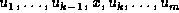are the children of y in. The value v is optional, and is assumed to be null if omitted.

Delete:
The deletion of a leaf node x of, denoted by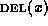. The resultis the same as, except that it does not contain node x.does not change the relative ordering of the remaining children of. This operation deletes only a leaf node; to delete an interior node, we must first move its descendents to their new locations or delete them.

Update:
The update of the value of a node x in, denoted by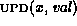.is the same asexcept that in,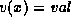.

Move:
The move of a subtree from one parent to another in, denoted by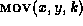.is the same as, except x becomes the kth child of y. The entire subtree rooted at x is moved along with x.

Figure 2 shows examples of edit operations on trees. In the figure, node 6 has label A and value foo. The labels and values of the other nodes are not shown.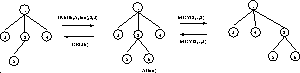: Edit operations on a treeNext: Edit Scripts Up: Overview and Preliminaries Previous: Overview and Preliminaries

Sudarshan S. Chawathe
Wed Jun 19 08:22:34 PDT 1996